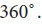Home | | Business Maths 11th std | Trigonometry: Introduction

# Trigonometry: Introduction

The study of trigonometry was first started in India. The ancient Indian Mathematician, Aryabhatta, Brahmagupta, Bhaskara I and Bhaskara II obtained important results.

Introduction

The word trigonometry is derived from the Greek word ‘tri’ (meaning three), ‘gon’ (meaning sides) and ‘metron’ (meaning measure). In fact, trigonometry is the study of relationships between the sides and angles of a triangle. Around second century A.D. George Rheticus was the first to define the trigonometric functions in terms of right angles. The study of trigonometry was first started in India. The ancient Indian Mathematician, Aryabhatta, Brahmagupta, Bhaskara  I  and  Bhaskara  II  obtained  important  results.

Bhaskara I gave formulae to find the values of sine functions for angles more than 90 degrees.The earliest applications of trigonometry were in the fields of navigation, surveying and astronomy.## Relations between trigonometric ratios## Angle

Angle is a measure of rotation of a given ray about its initial point. The ray ‘OA’ is called the initial side and the final position of the ray ‘OB’ after rotation is called the terminal side of the angle. The point ‘O’ of rotation is called the vertex.If the direction of rotation is anticlockwise, then angle is said to be positive and if the direction of rotation is clockwise, then angle is said to be negative.

## Measurement of an angle

Two types of units of measurement of an angle which are most commonly used namely degree measure and radian measure.

## Degree measureThe angle subtended at the centre of the circle by an arc equal to the length of the radius of the circle is called a radian, and it is denoted by 1c## Relation between degrees and radians

We know that the circumference of a circle of radius 1unit is 2 π. One complete revolution of the radius of unit circle subtends 2 π radians. A circle subtends at the centre an angle whose degree measure isTags : Definition, Formula, Solved Example Problems, Exercise | Mathematics , 11th Business Mathematics and Statistics(EMS) : Chapter 4 : Trigonometry
Study Material, Lecturing Notes, Assignment, Reference, Wiki description explanation, brief detail
11th Business Mathematics and Statistics(EMS) : Chapter 4 : Trigonometry : Trigonometry: Introduction | Definition, Formula, Solved Example Problems, Exercise | Mathematics